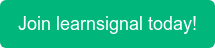##### What is Convexity?

Convexity relates to the interaction between a bond's price and its yield as it experiences changes in interest rates.

By measuring the change in duration as interest rates fluctuate, convexity, a measure of the curvature of changes in the price of a bond in proportion to changes in interest rates, corrects this inaccuracy.

Consider the case of convexity, which occurs when all spot rates change by the same amount.

##### Example of Convexity:

The formula is as follows:

C=d^2(Br)B*d*r^2

where

C=convexity

B=the bond price

r=the interest rate

d=duration

For example, consider a 200-basis-point increase in all rates for the bond in our example. The bond's price declines to USD 986,448.71 (a decrease in value of USD 51,473.32). Using duration alone indicates a price change of:

-2.56 * 1,037,922.03 * 0.02 = -53,141.61

But using the duration + convexity result it gives,

-53,141.61 + 1/2 * 8.246 * 1,037,922.03 *0.022 = -51,429.04

which is reasonably accurate.

Topics: ACCA, CIMA, CPD, AAT, FRM•### Central Counterparties

•### Country Risk

•### Convexity Formula

•### Clean and Dirty Price

•### Basic Indicator Approach

•### ACCA Exam Fees 2022: Everything You Need to Know

•### ACCA Exam Dates for 2022

•### How Difficult is Passing ACCA? An Honest Review

•### 7 of the Best YouTube Channels for Accounting Students

•